Miscellaneous

Chapter 7 Class 11 Binomial Theorem
Serial order wise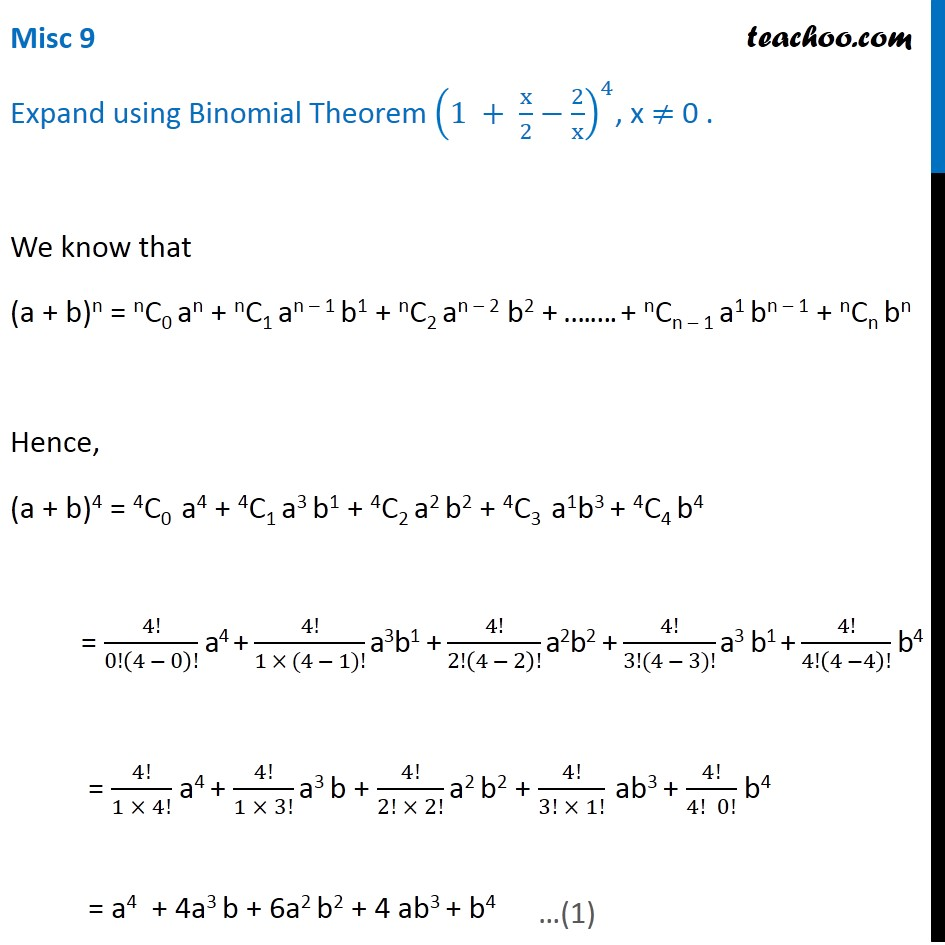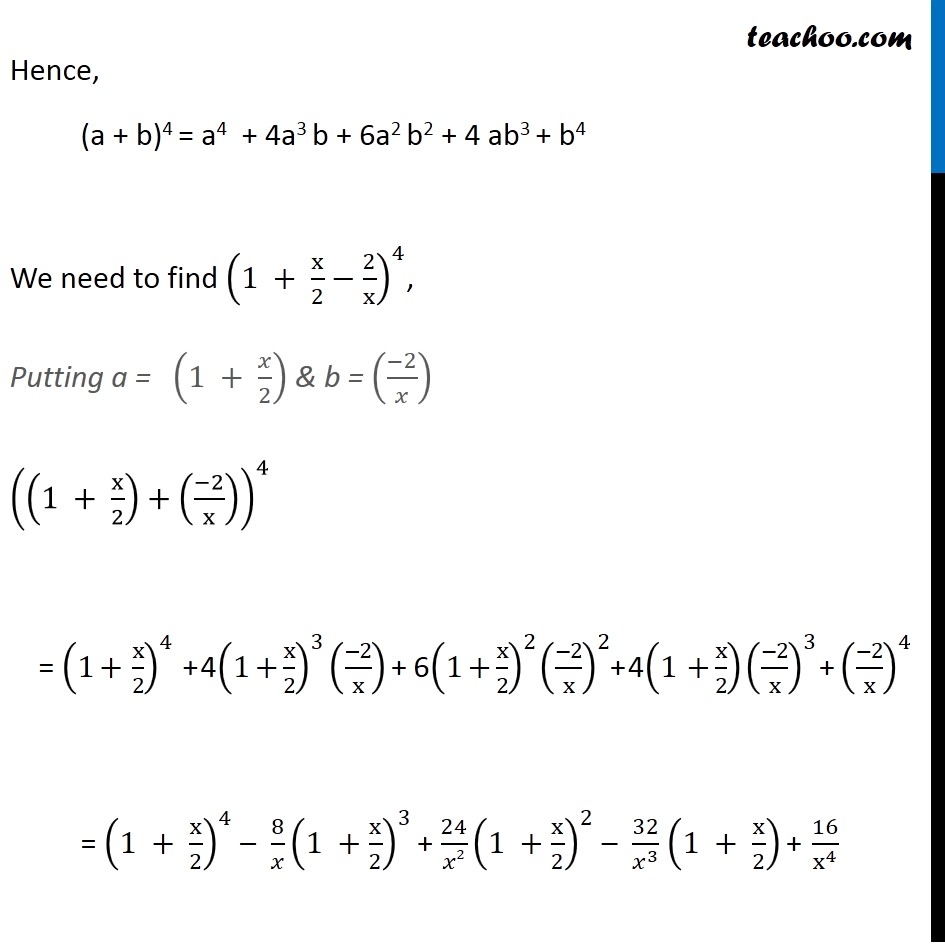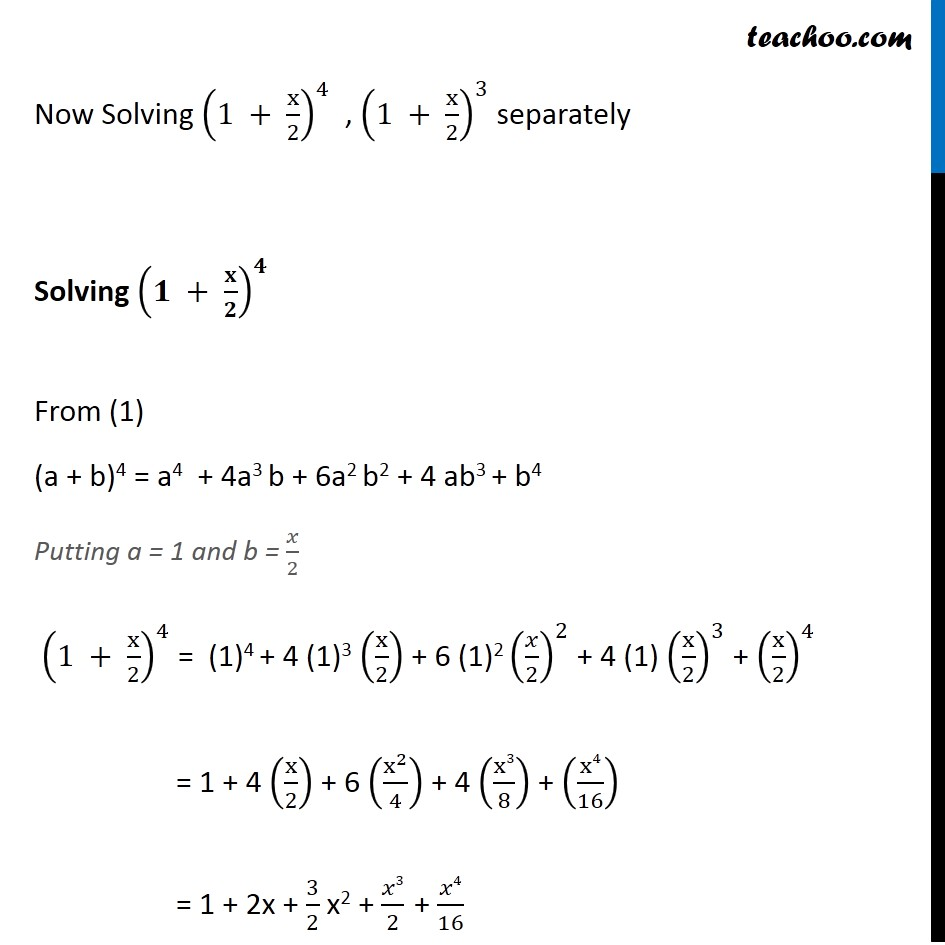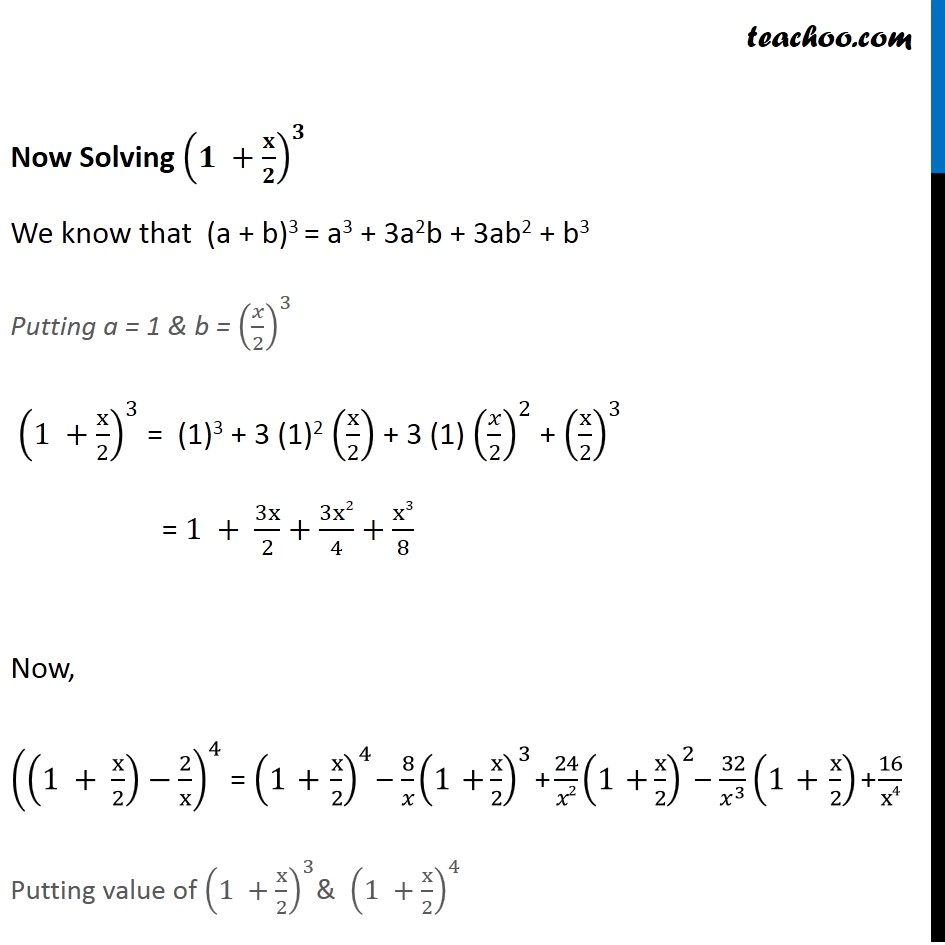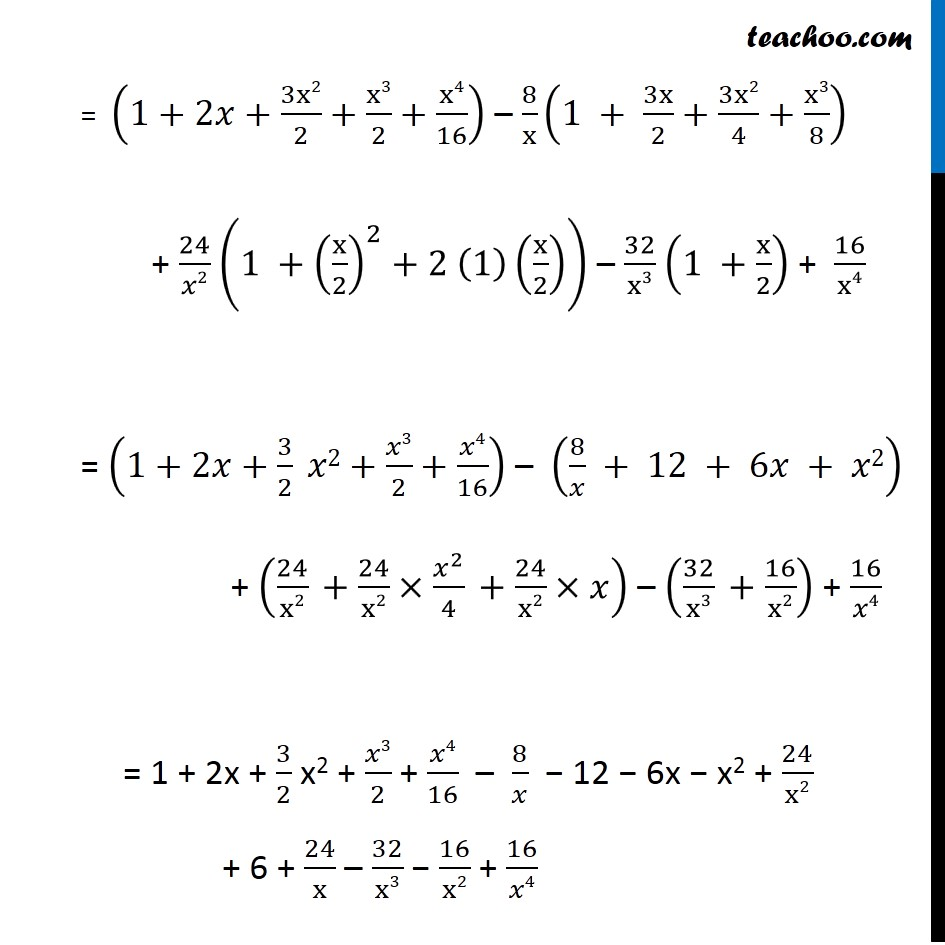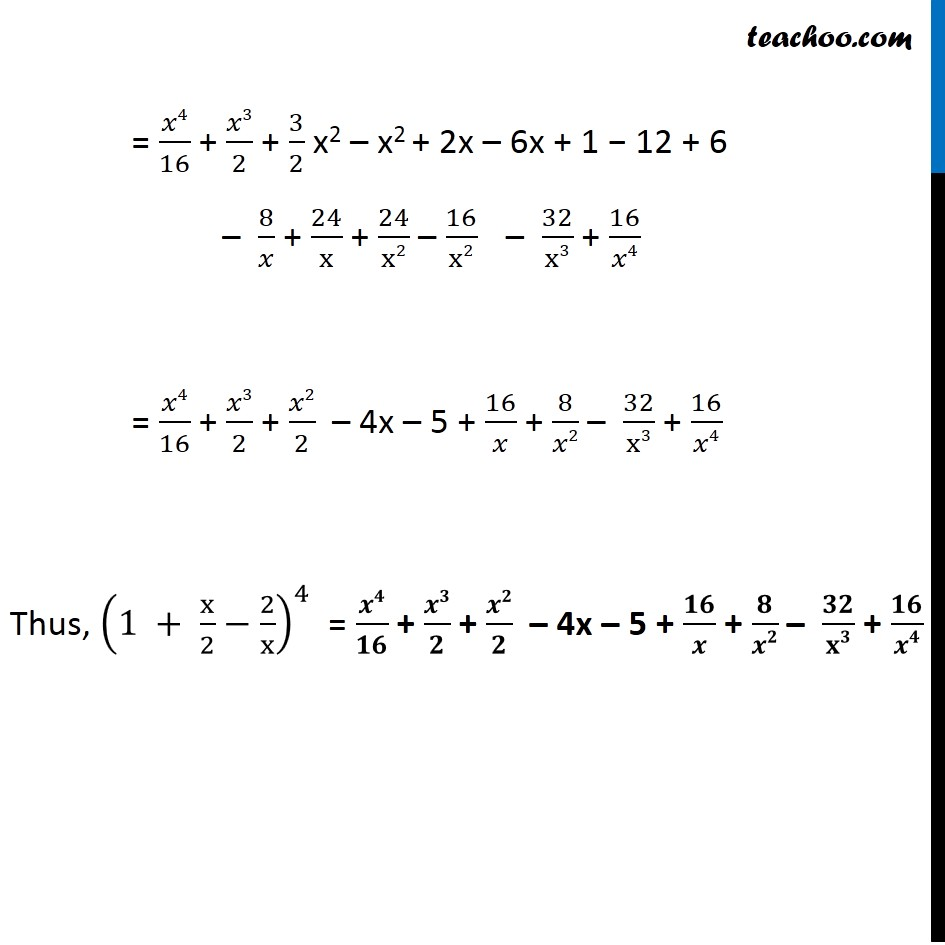Learn in your speed, with individual attention - Teachoo Maths 1-on-1 Class

### Transcript

Misc 5 Expand using Binomial Theorem (1 + x/2−2/x)^4, x ≠ 0 . We know that (a + b)n = nC0 an + nC1 an – 1 b1 + nC2 an – 2 b2 + ….…. + nCn – 1 a1 bn – 1 + nCn bn Hence, (a + b)4 = 4C0 a4 + 4C1 a3 b1 + 4C2 a2 b2 + 4C3 a1b3 + 4C4 b4 = 4!/0!(4 − 0)! a4 + 4!/(1 × (4 − 1)!) a3b1 + 4!/2!(4 − 2)! a2b2 + 4!/(3!(4 − 3)!) a3 b1 + 4!/4!(4 −4)! b4 = 4!/(1 × 4!) a4 + 4!/(1 × 3!) a3 b + 4!/(2! × 2!) a2 b2 + 4!/(3! × 1!) ab3 + 4!/(4! 0!) b4 = a4 + 4a3 b + 6a2 b2 + 4 ab3 + b4 Hence, (a + b)4 = a4 + 4a3 b + 6a2 b2 + 4 ab3 + b4 We need to find (1 + x/2−2/x)^4, Putting a = (1 + 𝑥/2) & b = ((−2)/𝑥) ((1 + x/2)+((−2)/x))^4 = (1+ x/2)^4 + 4 (1+x/2)^3 ((−2)/x) + 6 (1+x/2)^2 ((−2)/x)^2+ 4 (1 +x/2) ((−2)/x)^3 + ((−2)/x)^4 = (1 + x/2)^4 – 8/𝑥 (1 +x/2)^3 + 24/𝑥2 (1 +x/2)^2 – 32/𝑥^3 (1 + x/2) + 16/x^4 Now Solving (1 + x/2)^4 , (1 + x/2)^3 separately Solving (𝟏 + 𝐱/𝟐)^𝟒 From (1) (a + b)4 = a4 + 4a3 b + 6a2 b2 + 4 ab3 + b4 Putting a = 1 and b = 𝑥/2 (1 + x/2)^4 = (1)4 + 4 (1)3 (x/2) + 6 (1)2 (𝑥/2)^2 + 4 (1) (x/2)^3 + (x/2)^4 = 1 + 4 (x/2) + 6 (x^2/4) + 4 (x3/8) + (x4/16) = 1 + 2x + 3/2 x2 + 𝑥3/2 + 𝑥4/16 Now Solving (𝟏 +𝐱/𝟐)^𝟑 We know that (a + b)3 = a3 + 3a2b + 3ab2 + b3 Putting a = 1 & b = (𝑥/2)^3 (1 +x/2)^3 = (1)3 + 3 (1)2 (x/2) + 3 (1) (𝑥/2)^2 + (x/2)^3 = 1 + 3x/2+3x2/4+x3/8 Now, ((1 + x/2)−2/x)^4 = (1 + x/2)^4 – 8/𝑥 (1 +x/2)^3 + 24/𝑥2 (1 +x/2)^2– 32/𝑥^3 (1 + x/2) + 16/x4 Putting value of (1 +x/2)^3& (1 +x/2)^4 = (1+2𝑥+3x2/2+x3/2+x4/16) – 8/x (1 + 3x/2+3x2/4+x3/8) + 24/𝑥2 (1 +(x/2)^2+2 (1)(x/2)) – 32/x3 (1 +x/2) + 16/x4 = (1+2𝑥+3/2 𝑥2+𝑥3/2+𝑥4/16) – (8/𝑥 + 12 + 6𝑥 + 𝑥2) + (24/x2 +24/x2×𝑥^2/4 +24/x2×𝑥) – (32/x3 +16/x2) + 16/𝑥4 = 1 + 2x + 3/2 x2 + 𝑥3/2 + 𝑥4/16 – 8/𝑥 − 12 − 6x − x2 + 24/x2 + 6 + 24/x – 32/x3 − 16/x2 + 16/𝑥4 = 𝑥4/16 + 𝑥3/2 + 3/2 x2 – x2 + 2x – 6x + 1 − 12 + 6 – 8/𝑥 + 24/x + 24/x2 – 16/x2 – 32/x3 + 16/𝑥4 = 𝑥4/16 + 𝑥3/2 + 𝑥2/2 – 4x – 5 + 16/𝑥 + 8/𝑥2 – 32/x3 + 16/𝑥4 Thus, (1 + x/2−2/x)^4 = 𝒙𝟒/𝟏𝟔 + 𝒙𝟑/𝟐 + 𝒙𝟐/𝟐 – 4x – 5 + 𝟏𝟔/𝒙 + 𝟖/𝒙𝟐 – 𝟑𝟐/𝐱𝟑 + 𝟏𝟔/𝒙𝟒